OEImage¶

class OEImage : public OEImageBase

OEImage class is implemented as a display list i.e. a container of drawing commands. For example, when the OEImage.DrawLine method is called, rather than immediately drawing a line specified by the parameters, a line drawing operation is created that copies all parameters for later execution.

When the OEImage.Render method is invoked, the drawing commands stored in the OEImage object are executed in the same order in which they were issued.

The following methods are publicly inherited from OEImageBase:

Constructors¶

OEImage(double width, double height, const OESystem::OEColor& bgColor = OESystem::OEWhite);

Default constructor that creates an OEImage with the specified width and height.

width, height

The dimensions of the image, both have to be positive (non-zero) numbers.

bgColor

The color that is used to clear the image upon construction. The default background color is OEWhite. A .png image with transparent background can be generated by passing the OETransparentColor as the background color.

OEImage(const OEImage &rhs)

Copy constructor.

OEImage(const OEImage &src, double scale)

Copy constructor with scaling.

scale

The scaling factor is used to create a new image from the given one. The scaling factor has to be a positive (non-zero) number.

The following code snippet shows how to half and double the size of an image.

OEImage image = new OEImage(100.0, 100.0);

image.DrawCircle(OEDepict.OEGetCenter(image), 40.0, OEDepict.OEBlackPen);
image.DrawText(OEDepict.OEGetCenter(image), "circle", OEDepict.OEDefaultFont);
OEDepict.OEDrawBorder(image, OEDepict.OELightGreyPen);

OEDepict.OEWriteImage("image.png", image);

OEImage halfimage = new OEImage(image, 0.5);
OEDepict.OEWriteImage("halfimage.png", halfimage);

OEImage doubleimage = new OEImage(image, 2.0);
OEDepict.OEWriteImage("doubleimage.png", doubleimage);
Examples of image scaling

original image

scale = 0.5

scale = 2.0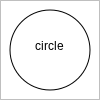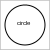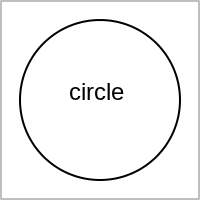Clear¶

void Clear(const OESystem::OEColor &color)

Appends a clear command to the display list of the OEImage object.

DrawArc¶

void DrawArc(const OE2DPoint &center, double bgnAngle, double endAngle,

Appends an arc drawing command to the display list of the OEImage object. See example in Figure: Example of drawing an arc.

DrawCircle¶

void DrawCircle(const OE2DPoint &center, double radius, const OEPen &pen)

Appends a circle drawing command to the display list of the OEImage object. See example in Figure: Example of drawing a circle.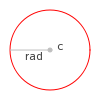Example of drawing a circle

DrawCubicBezier¶

void DrawCubicBezier(const OE2DPoint &bgn, const OE2DPoint &c1,
const OE2DPoint &c2, const OE2DPoint &end, const OEPen &pen)

Appends a cubic Bézier curve drawing command to the display list of the OEImage object. See example in Figure: Example of drawing a cubic Bezier curve.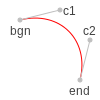Example of drawing a cubic Bezier curve

DrawLine¶

void DrawLine(const OE2DPoint &bgn, const OE2DPoint &end, const OEPen &pen)

Appends a line drawing command to the display list of the OEImage object. See example in Figure: Example of drawing a line.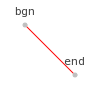Example of drawing a line

DrawPath¶

void DrawPath(const OE2DPath& path, const OEPen& pen);

Appends a path drawing command to the display list of the OEImage object. See example in Figure: Example of drawing a path.

DrawPie¶

void DrawPie(const OE2DPoint &center, double bgnAngle, double endAngle,

Appends a pie drawing command to the display list of the OEImage object. See example in Figure: Example of drawing a pie.

DrawPoint¶

void DrawPoint(const OE2DPoint &p, const OESystem::OEColor &color)

Appends a point drawing command to the display list of the OEImage object. See example in Figure: Example of drawing a point.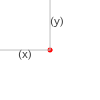Example of drawing a point

DrawPolygon¶

void DrawPolygon(const std::vector<OE2DPoint> &points, const OEPen &pen)

Appends a polygon drawing command to the display list of the OEImage object. See example in Figure: Example of drawing a polygon.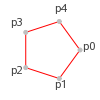Example of drawing a polygon

void DrawQuadraticBezier(const OE2DPoint &bgn, const OE2DPoint &c,
const OE2DPoint &end, const OEPen &pen)

Appends a quadratic Bézier curve drawing command to the display list of the OEImage object. See example in Figure: Example of drawing a quadratic Bezier curve.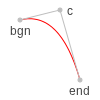Example of drawing a quadratic Bezier curve

DrawRectangle¶

void DrawRectangle(const OE2DPoint &tl, const OE2DPoint &br, const OEPen &pen)

Appends a polygon drawing command to the display list of the OEImage object. See example in Figure: Example of drawing a rectangle.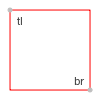Example of drawing a rectangle

DrawText¶

void DrawText(const OE2DPoint &c, const std::string &text, const OEFont &font,
double maxwidth=0.0)

Appends a text drawing command to the display list of the OEImage object. See example in Figure: Example of drawing a text.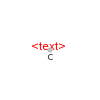Example of drawing a text

DrawTriangle¶

void DrawTriangle(const OE2DPoint &a, const OE2DPoint &b, const OE2DPoint &c,
const OEPen &pen)

Appends a triangle drawing command to the display list of the OEImage object. See example in Figure: Example of drawing a triangle.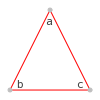Example of drawing a triangle

GetHeight¶

double GetHeight() const

Returns the height of the OEImage object.

GetWidth¶

double GetWidth() const

Returns the width of the OEImage object.

IsEmpty¶

bool IsEmpty() const

Returns whether or not the display list of the OEImage object is empty.

Render¶

bool Render(OEImageBase &image) const

Renders the image stored in the OEImage object by executing the stored drawing commands in the order in which they were issued.